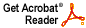# Zeros of random functions in Bergman spaces

Joel H. Shapiro

Ann. Inst Fourier (Grenoble) 29 (1979), 159--171

Abstract: Let mu be a finite positive rotation-invariant Borel measure on the unit disc, whose support goes out to the boundary, and let (A^p)_mu denote the weighted Bergman spaces associated with mu. In a previous paper [Zeros of functions in weighted Bergman spaces, Michigan Math. J. 24 (1977), no. 2, 243 256] I proved that for p and q different, the zero sets of functions in (A^p)_mu are different from those of the functions in (A^q)_mu. Here I show, among other things, that a Gaussian (random) power series which almost surely lies in (A^p) _mu but not in (A^q) _must almost surely have an (A^p) _mu zero set which is not an (A^q)_mu zero set. The proof is based on an idea of Walter Rudin [Nederl. Akad. Wetensch. Proc. Ser. A 38 (1976), 57--65], together with some elementary probability theory.
Download .pdf file (748KB)